What's New in Phase Diagram

What follows are summaries of the more important programming changes in Phase Diagram that have appeared in:

FactSage 6.1 (2009)

• In the Menu Window you save the Phas*.dat file by clicking on 'File > Save as ...'. If the phase diagram has just been calculated you now have the option of saving the calculated figure (including any added labels, tie-lines, etc.) either within the Phas*.dat file or in a Phas*.fig file that has the same name (Phas*) but .fig extension. In either case, the next time you load the Phas*.dat file that has such a stored figure, you will be prompted 'Show the figure calculated last time?'.

• In the Variables Window (click on 'Variables' in the Menu Window) it is now possible to calculate and plot polythermal projections of the liquidus surfaces of ternary (and reciprocal salt) systems - in the 'Temperature Frame' select 'projection' from the combo menu (the other options are 'Y-axis', 'X-axis' and 'constant'). For both ternary Gibbs phase diagram and X versus Y reciprocal systems, the polythermal projection of the liquidus surfaces will appear as a series of isothermal lines and univariant lines (where the liquidus surfaces intersect). You can specify the upper and lower temperatures of the isothermal sections (or ask the program automatically calculate them for you) as well as the step in temperature.

• In the Parameters Window (click on 'Parameters' in the Menu Window) new options are available for the display of the polythermal projections. For example, the minimum and maximum temperatures and all invariant points (compositions and temperatures) on the liquidus surface as well as the names of the intersecting phases can be tabulated. The projections may be color coded by temperature (hot 'red' colors at high temperatures down to cold 'blue' colors at low temperatures) or by phase.

The appearance of the calculated diagram is greatly influenced by the settings in this screen. The projections may for example be colour coded by temperature (hot 'red' colours at high temperatures down to cold 'blue' colours at low temperatures) or by phase. See below

• In plotted figure (click on 'Calculate' in the Menu Window) the optional status box now includes the time step (seconds) between calculations.

• After the phase diagram has been calculated, for polythermal projections the liquidus temperature at any composition (together with its precipitating phase) is automatically displayed by simply pointing the mouse to the appropriate coordinate on the figure.

• Sample polythermal projections using FACT data may be loaded and displayed - click on 'Open > Change Directory' and select 'Polythermal Projections'. Alternatively, the calculated phase diagrams can also be displayed using the Figure module - the figures are stored as *.fig files in the \FIGURES folder of FactSage.

• The Slide Show has been edited to reflect new additions to FactSage 6.1 including Gibbs ternary polythermal projections of CaCl2-LiCl-KCl and Al2O3-CaO-SiO2, and a reciprocal salt polythermal projection of CaCl2-NaF-CaF2-NaCl.

FactSage 6.2 (2010)

• Non-Stoichiometric Compounds, FToxid-SLAG Information, Miscibility Gap - Option 'I'
- Please refer to the Equilib section above for the programming changes that are common to both Equilib and Phase Diagram.

• Gas Surface Projection - It is now possible to plot the polythermal projections of condensation and of evaporation surfaces. Examples of gas surface projections have been added in the Phase Diagram Slide Show.

• Enthalpy-Composition Diagram - It is now possible to calculate enthalpy-composition (H-X) phase diagrams. The vertical axis is the enthalpy relative to the enthalpy at a specified reference temperature (for example, 25oC) and the horizontal axis is the composition, either in a binary system or along a constant composition path (isopleth) in a multicomponent system.

The enthalpy-composition (H-X) diagram is defined in the Variables Window. In the Temperature frame you select 'enthalpy' from the drop-down menu and then you define enthalpy-maximum, temperature-minimum and the step in temperature. It is recommended that you select the option to plot the 'iso-thermal lines'.

The resulting diagram permits you to read at a glance the heat change associated with each stage of cooling a mixture (sensible heat during cooling a single phase, heat change during eutectic reactions, etc.) . An example of a enthalpy-composition diagram has been added to the Phase Diagram Slide Show.

• Parameters Window - The Phase Diagram graphical settings that are displayed in the Parameters Window have been expanded and reformatted.

• Labels & Lines - the line color, line width and line style are new.

• Iso-Thermal Section - the enthalpy line color and enthalpy line style are new.

• Polythermal Projection - this now includes an option to automatically label all the projection surfaces (the manual mouse 'point and click' is still available). Also there is an option to label the intersection points with a number # (as before) or with the actual temperature (this is new) using a user-defined color (this is new).

• Units - The units listed on the X and Y axes are now reported as 'grams/grams' and 'mol/mol'. These are more correct than 'mass' and 'mole' that were reported prior to FactSage 6.2.

FactSage 6.3 (2012)

1 Parameters Window
- called from 'Menu Window > Parameters'.

 1.1 Beep when finished - it is now possible to issue a sound ('friendly beep' unique to Phase Diagram) when a calculated phase diagram is finished. This is useful in time consuming calculations when you want to know the moment the resulting diagram is ready.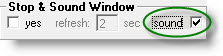1.2. Intersection points - for polythermal projections, tabulated lists of intersecting phases, compositions and temperatures may be displayed on the calculated figure, or in a separate text window, or both.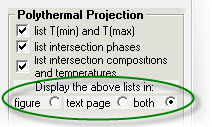Screenshot showing tabulated lists both on the calculated figure and in a separate text window for the case of the CaCl2-LiCl-KCl liquidus projection.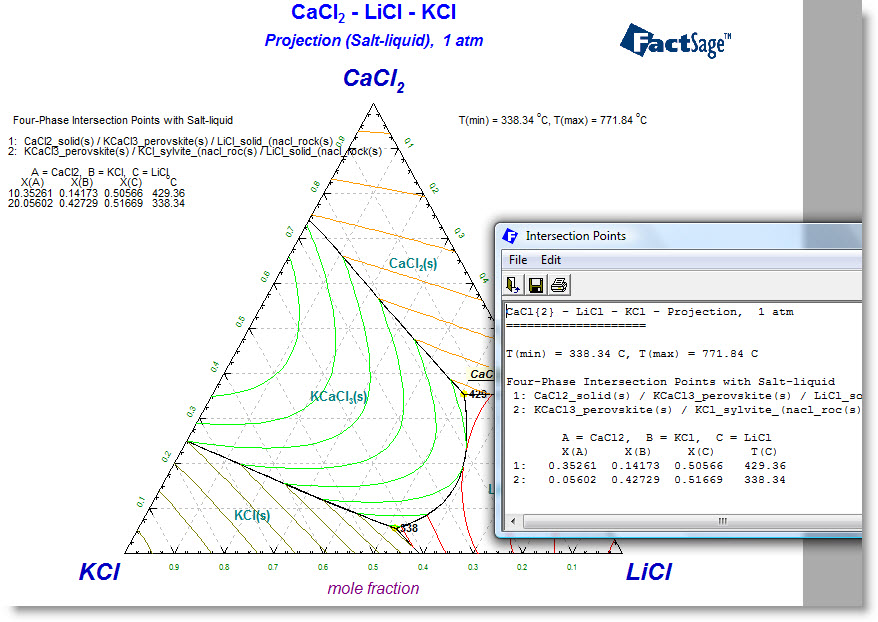2 Variables Window
- called from 'Menu Window > Variables'.

2.1. Volume diagrams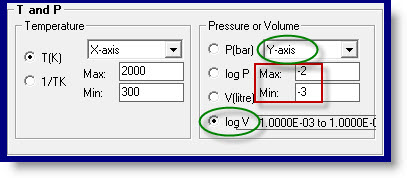'Volume' or 'log volume' may be specified as an X- or Y-axis. Typical volume diagrams include 'V (or log V) versus T' and 'V (or log V) versus X'.

Screenshot of log(V) versus T(K) in the Si-C-O system when Si/(Si+C+O) = 0.1 (mol/mol) and C/(Si+C+O) = 0.3 (mol/mol).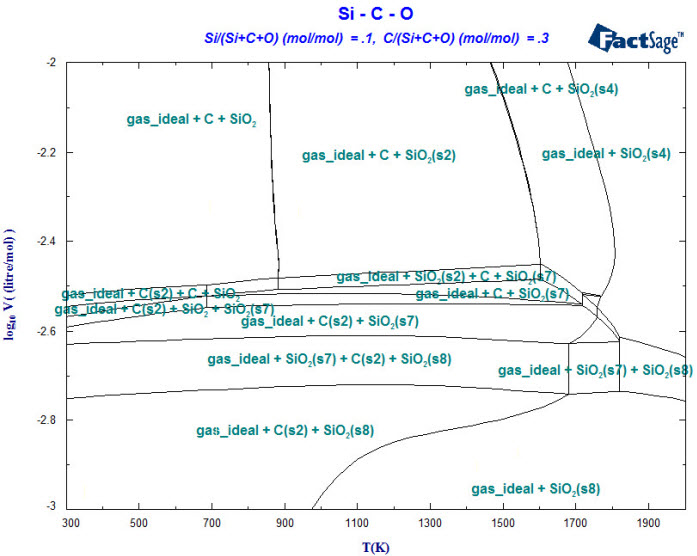2.2. Polythermal Projections

 2.2.1 Alloy Liquidus Projection from the SGTE 2011 Databases Polythermal Projections were first introduced in FactSage 6.1. It is possible to calculate and plot polythermal projections of surfaces (typically the liquidus surface) of ternary (or reciprocal salt) systems. In the 'Temperature Frame' select 'projection' from the combo menu as shown in the screenshot. The screenshot shows option "Projection" selected in the Variables Window.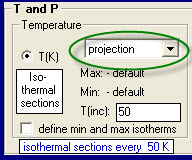In the Menu Window it is then necessary to identify the target solution phase - typically a liquid solution (e.g. FToxid-slag, FTsalt-liquid) in the case of a liquidus projection. This is done by assigning option "O" (Only plot this phase) for the target solution phase being selected. In FactSage 6.3 you can alternatively assign option "P" (precipitate target phase). That is, options "O" and "P" are equivalent. Liquidus projection - the screenshot shows option "P" assigned to the SGTE-liquid phase (e.g. mouse-right-click on the '+' column).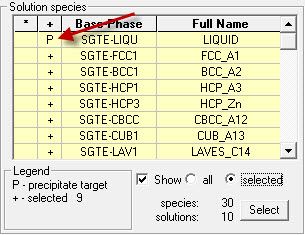For both ternary Gibbs phase diagram and X versus Y reciprocal systems, the polythermal projection of the liquidus surfaces will appear as a series of isothermal lines and univariant lines where the liquidus surfaces intersect.

The screenshot of the polythermal projection of the Ca-Mg-Sn liquidus surface.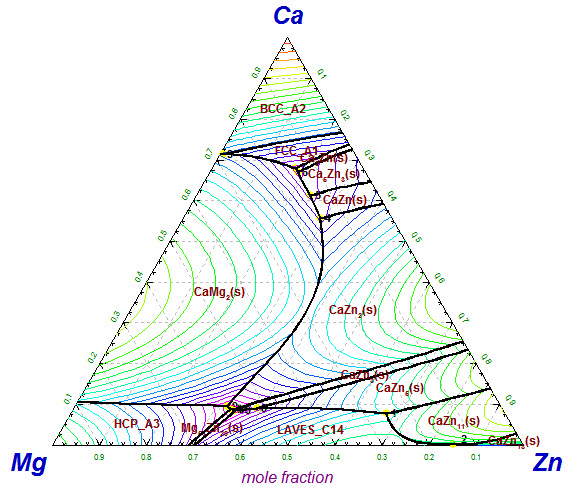2.2.2 Alloy First Melting Projection from the SGTE 2011 Databases It is now possible to calculate and plot polythermal projections of a formation surface (typically the first melting or solidus surface) of ternary (or reciprocal salt) systems. In the 'Temperature Frame' of the Variables Window elect 'projection' from the combo menu as before. In the Menu Window it is then necessary to identify the formation target solution phase - typically a liquid solution (e.g. FToxid-slag, FTsalt-liquid) in the case of a first melting or solidus projection. This is done by assigning option "F" (formation target phase) for the target solution phase being selected. First melting projection - the screenshot shows option "F" assigned to the SGTE-liquid phase (e.g. mouse-right-click on the '+' column)The screenshot of the polythermal projection of the Ca-Mg-Zn first melting surface.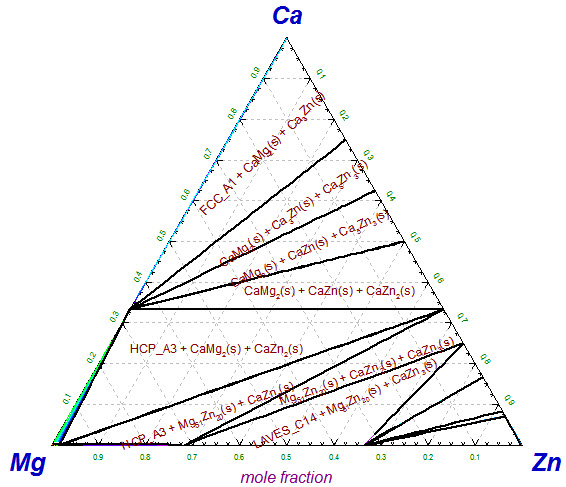2.3 Oxide Liquidus and First Melting Projections from the FToxid databases

Screenshots of the polythermal projection of the Fe2O3-Al2O3-SiO2 liquidus surface (left) and first melting surface (right).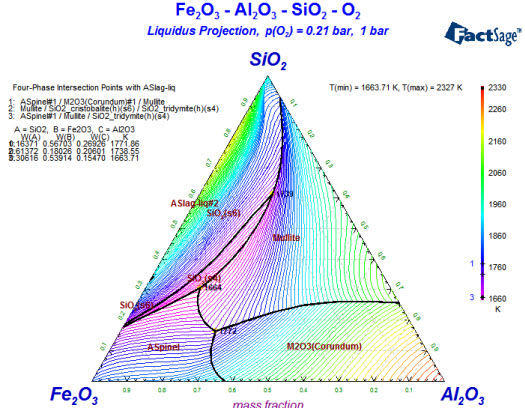FactSage 6.4 (2013)

In FactSage 6.4 when you first run Phase Diagram then a summary of 'Current Databases Selected' and 'Current Data Search Options' is posted and you are asked if you want to 'Load last system ?'.

If you reply 'Yes' then the last Phase Diagram system is loaded as in previous versions.

If you reply 'No' you now have the the option to keep the current 'Databases Selected' and 'Data Search Options', or, clear the 'Databases Selected', apply default settings to 'Data Search Options' and open the 'Data Search Window'."

 2 Compound Species Selection - see Slide Show 'Phase Diagram - Section 3.2.1' There is a new default exclusion of species from compound species selection When two or more databases are connected, the same species may appear in more than one database. In such cases, a species should generally only be selected from one database. Otherwise conflicts will probably occur. In order to assist users in deciding which species to exclude, the FactSage developers have assigned priorities. When you initially click on "pure solids", "pure liquids", or "gas" you may now see that several species marked with an "X" have not been selected. That is, they have been excluded by default because of probable conflicts between databases. The FactSage developers suggest that these species not be selected for this particular calculation. If you wish to select species marked with an "X" you must first click on 'permit selection of "X" species'. This will then override the default setting and permit you to select species as in FactSage This will also activate the 'suppress duplicates' button and enable you to define a database priority list as in FactSage IMPORTANT : For many calculations, it may frequently be advisable or necessary to de-select other species in addition to those marked with an "X." - refreshing compound default selection - This message will appear whenever you change the selected databases, or change the 'Data Search Options', or change the components; that is any action that requires the databases to be re-scanned. The purposr of the message is to simply advise you that the compound species selectiom may have changed. The message will not appear when you load a new system or when you load a stored file.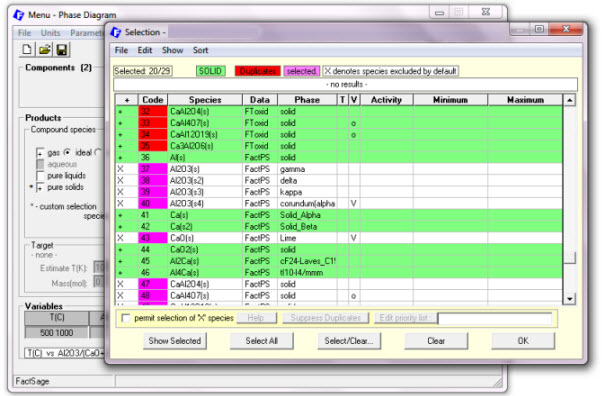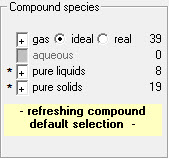3 Enhanced phase diagram pictographsIn the Menu Window the small pictographs of the Gibbs ternary triangle and the reciprocal system square now include the chemical formulae of the system components. The X vs Y binary pictograph remains unchanged.

 4. Paraequilibrium diagrams - see Slide Show 'Phase Diagram - Section 15' Paraequilibrium refers to the concept that diffusion kinetics of interstitial solutes is much faster than that of substitutional solutes. For example in the Fe-Cr-C system carbon diffuses between solid phases much more rapidly than Fe and Cr. That is, the diffusion of Fe and Cr between solid phases can be ignored. A new paraequilibrum checkbox that performs paraequilibrium diagrams is now offered in the Phase Diagram Menu Window . In addition an Edit button opens an input box where the diffusing solutes (typically elemental carbon) are defined.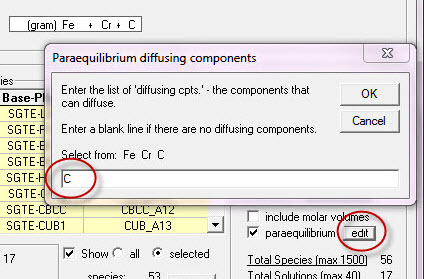For example, the first figure below is the T(C) versus Wt.% C phase diagram of the Fe-Cr-C system at 2.8 Wt.% Cr calculated under normal equilibrium conditions (orthoequilibrium). Data are taken from the SGTE 2011 databases.

The second figure is the paraequilibrium diagram for the same system when C is the only diffusing component.

For a given system the paraequilibrium diagram tends to be simpler in appearance than the orthoequilibrium diagram. For example the first figure is a ternary phase diagram. With the constraint of paraequilibrium and carbon diffusing the second figure is a binary phase diagram. If no species are permitted to diffuse the system would be reduced even further into a unary phase diagram (not shown here).

It is also possible to calculate phase diagrams in which the phase fields show the single phase with the minimum Gibbs energy at any given point on the diagram. Such calculations may be of practical interest in physical vapour deposition where deposition from the vapour phase is so rapid that phase separation cannot occur, resulting in a single-phase solid deposit.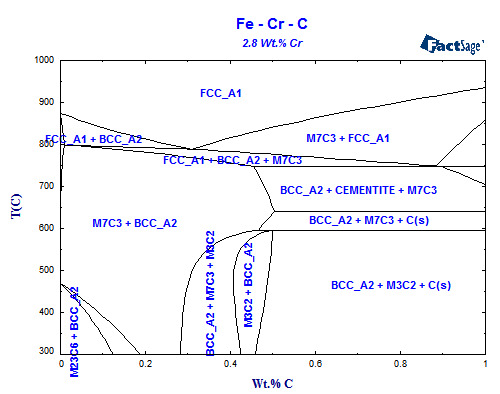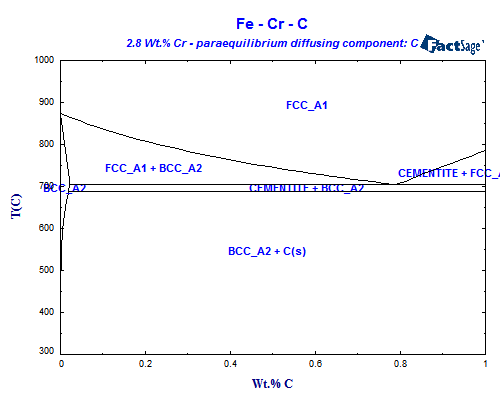5 Reciprocal diagrams CaCl2-NaF-CaF2-NaCl is a reciprocal salt system because the chemistry can be defined by the following exchange reaction: CaCl2+ 2NaF = CaF2+ 2NaCl The components are Na, Ca, F, and Cl. The Y-axis is : F /(F + Cl): 0 to 1 The X-axis is : 2Ca /(F + Cl): 0 to 1 The constant is : (2Ca + Na) /(F + Cl) = 1 This forces electroneutrality since moles: 2n(Ca[++]) + n(Na[+]) = n(F[-]) + n(Cl[-]) The x-axis should be 2Ca /(2Ca + Na) but (2Ca + Na) = (F + Cl) The diagram is not the CaCl2-NaF-CaF2-NaCl system but rather CaCl2-(NaF)2-CaF2-(NaCl)2 Entry of these variables using the Variables Window is documented in the slide show. However the entries are cumbersome and not so obvious. In addition the resulting diagram is missing information about the corners: CaCl2-(NaF)2-CaF2-(NaCl)2.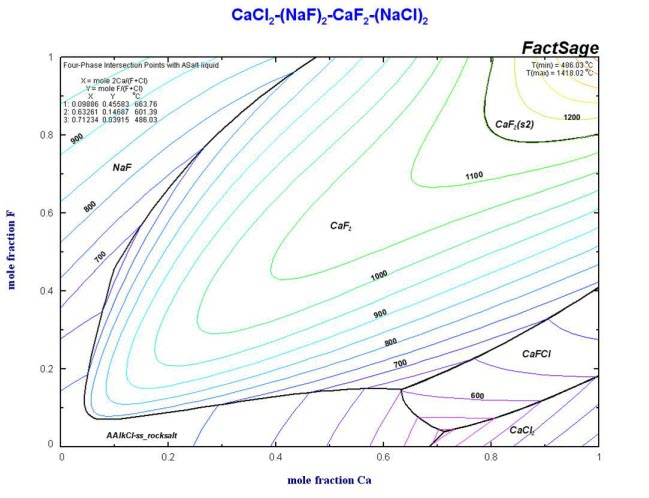6 New reciprocal diagram option In FactSage 6.4 there is a new option for calculating reciprocal diagrams. In the Components Window when you check the 'reciprocal diagram' checkbox you are offered a new menu where you simply enter the 2 cationic and 2 anionic components and associated charges.This automatically activates the correct 'reciprocal settings' in the Variables Window. It is necessary to define the temperature (or select 'projection') and the pressure (typically '1'). No further editing of the axes is required. The resulting calculated diagram is a true CaCl2-(NaF)2-CaF2-(NaCl)2 reciprocal system with a 'square frame' and the corners labelled accordingly.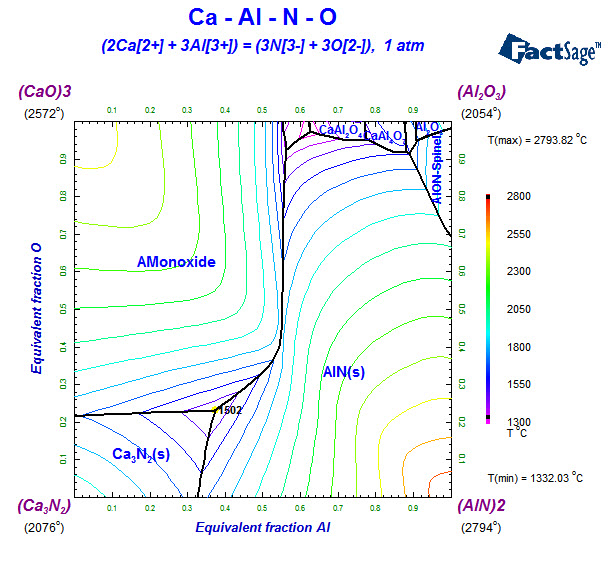Reciprocal diagrams are not limited to molten salts Here is the (CaO)3-Al2O3-Ca3N2-(AlN)2 reciprocal diagram with data taken from the FTOxCN database.7 New first melting projection and solidus projections - see Slide Show 'Phase Diagram - Sections 13.5 - 13.10' The slide show includes new examples of catatectics or retrograde solubility, solidus projections and first melting temperature projections To display the polythermal projections in Phase Diagram click on 'File > Directories > Polythermal Projections ... '. In the Directory Phase Diagram Window make sure that 'Tools > Show Preview Diagram' is checked.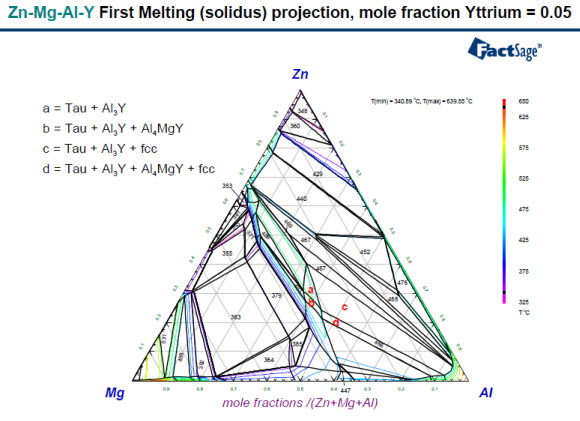8 Phase Diagrams with Various X- and Y-axes

In a Phase Diagram, two thermodynamic properties are chosen as X- and Y-axes, while other properties are held constant. The properties that may be selected as axes or constants are:

T (or 1/TK) - temperature; P - total pressure; V - volume; Comp - Composition (mole fractions, molar ratios, weight fractions, weight ratios); Potl - Chemical potentials (RT ln(ai), RT ln(Pi), log(ai), log(Pi) where ai & Pi = activity or partial pressure of component i); Delta H - Enthalpy relative to a standard state temperature; E, pH - Potential (volts) and pH axes of an aqueous Pourbaix-type diagram.

Not all combinations are permitted. For example, T (Y-axis) vs Comp (X-axis) is permitted, but not Comp (Y-axis) vs T (X-axis). The following table summarizes the various combinations of variables that may be selected in Phase Diagram as X- and Y-axes and as constants.

 Type Y-axis X-axis Constants T vs Comp T Comp P Comp vs Comp Comp Comp P, T Potl vs Comp Potl Comp P, T Potl vs T (or T vs Potl) PotlT TPotl PP Potl vs Potl Potl Potl P, T P vs T (or T vs P) PT TP V vs T (or T vs V) VT TV V vs Comp V Comp T P vs Potl (or Potl vs P) PPotl PPotl TT Comp vs Comp Comp Comp P, T Delta H vs Comp Delta H Comp T, P, molalities Gibbs Triangle Diagrams T, P Projection Diagrams Paraequilibrium Diagrams Minimum Gibbs Energy Diagrams

Approximately 50 example diagrams with various X- and Y-axes are stored in Phase Diagram. To load and calculate the example diagrams, run Phase Diagram and click on 'File > Directories > Phase diagrams with various......'. This list of examples is by no means exhaustive.

FactSage 7.0 (2015)

1 Enhanced detailed point calculation

 After a diagram has been displayed, you calculate the equilibrium at any position on the diagram by selecting the 'phase equilibrium mode', and then pointing and clicking at the desired spot. In FactSage 7.0, after clicking you now have the option to enter the precise coordinates for the detailed point calculation. The screenshot shows the calculated equilibrium in the Cu-O binary system at 1300oC and XO = 0.35 using data from the public FScopp database.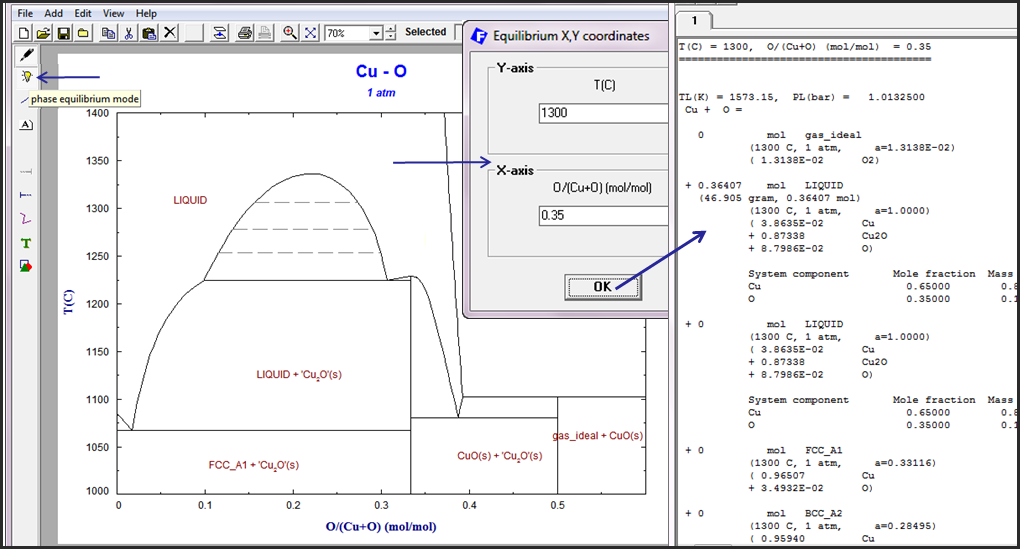2 Isobars and iso-activity lines - new option 'Z'

In FactSage 7.0 you can plot isobars of a gas species or gas phase and iso-activity lines of a compound (liquid, solid).

For a compound species the isobar or iso-activity is defined in the Selection Window (activated in the Menu Window by a mouse-right-click on a 'Compound species' check box). In the Selection Window mouse-right-click on the selected species, select 'Z isobars..' and then enter the values.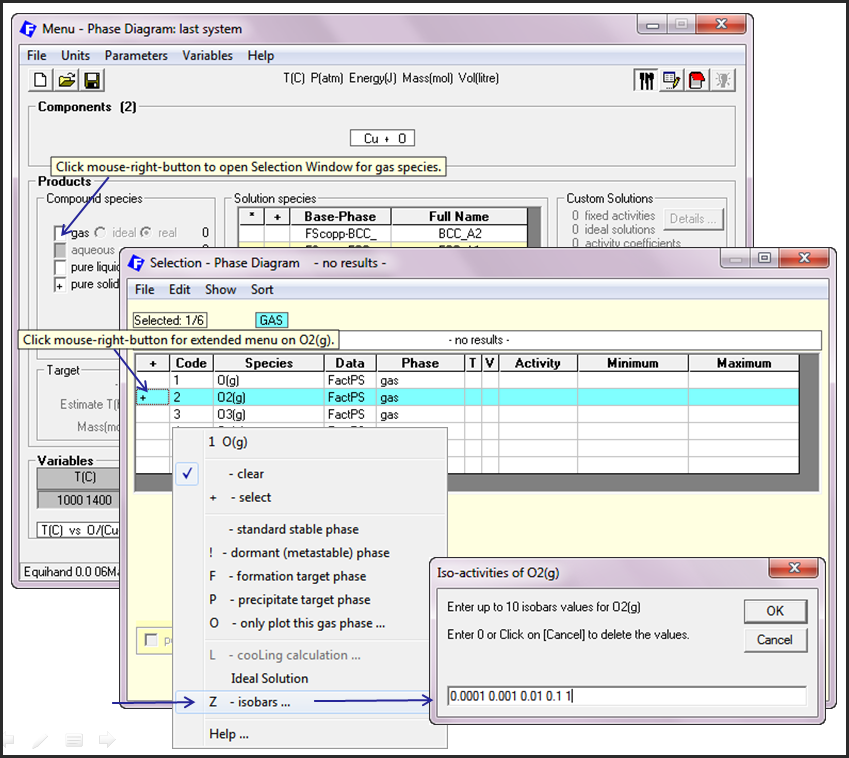The screenshots show the calculation of O2(g) isobars in the Cu-O binary systems using data from the public FScopp database.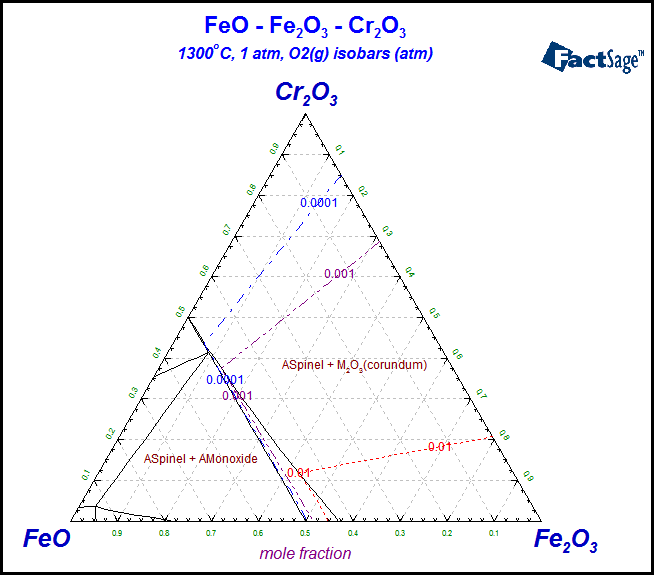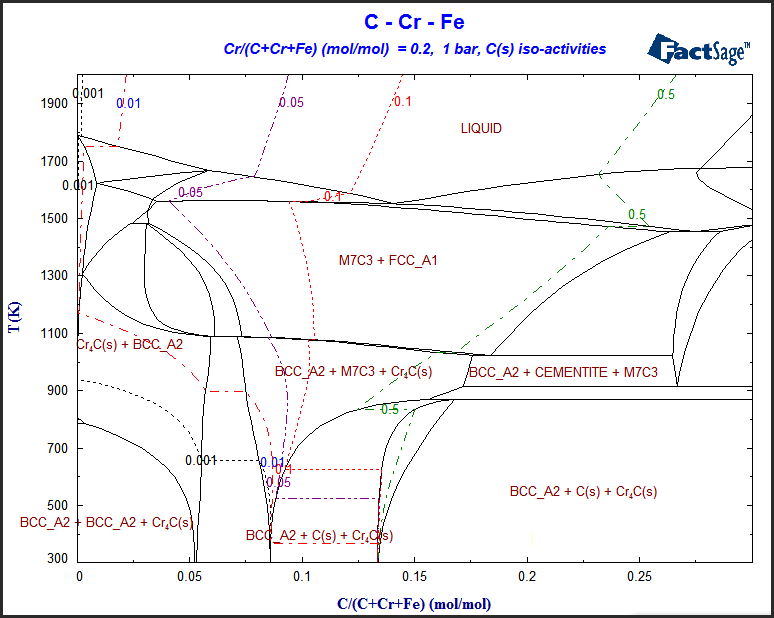Left: calculation of O2(g) isobars in the FeO-Fe2O3-Cr2O3 ternary system at 1300oC using data from the public FToxid database.
Right: calculation of C(s) iso-activity lines in the Fe-Cr-C ternary system using data from the public SGTE2011 database.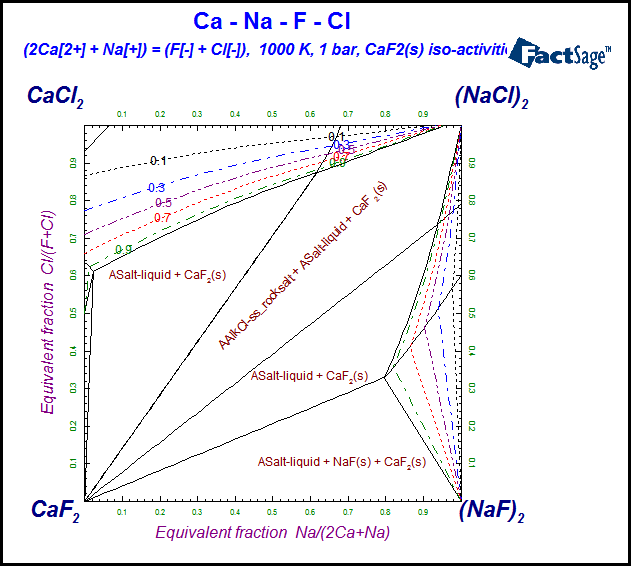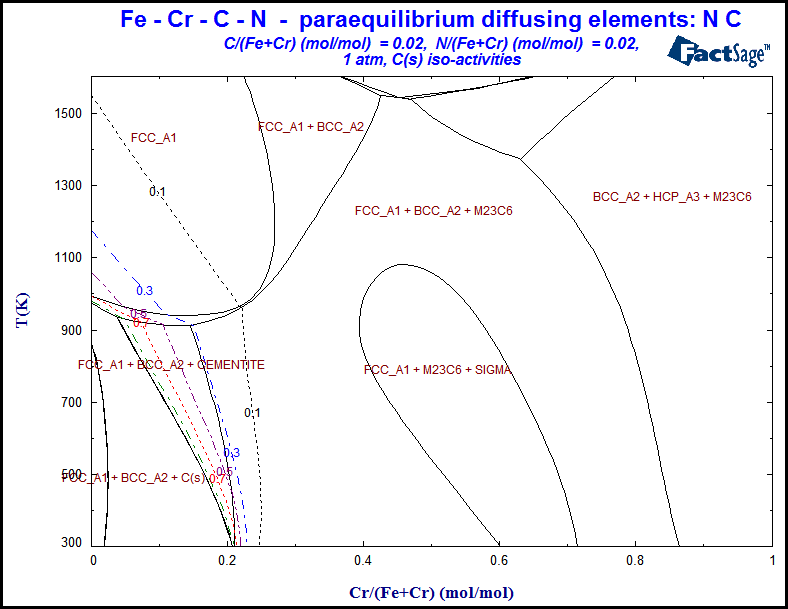Left: calculation of CaF2(s) iso-activities in CaF2-NaCl-CaCl2-NaF reciprocal salt system at 1000 K using data from the public FTsalt database.
Right: calculation of C(s) iso-activities in Fe-Cr-C-N paraequilibrium diagram with diffusing elements N and C using data from the public SGTE2011 database.

Approximately 60 example diagrams with various X- and Y-axes are stored in Phase Diagram. To load and calculate the phase diagrams with isobars and iso-activity lines that are shown above run Phase Diagram and click on 'File > Directories > Phase diagrams with various......'. This list of examples is by no means exhaustive.

FactSage 7.1 (2017)

1. Phase Diagram Components Window

• New types of phase diagrams  It is now possible to calculate Scheil-Gulliver Constituent Diagrams and Aqueous Phase Diagrams. Details are given below in the section 3. Phase Diagram Variables Window.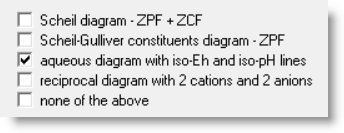• Classical Eh-pH (Pourbaix) diagrams no longer calculated

Classical Eh-pH (Pourbaix) diagrams are not true phase diagrams and have been replaced by Aqueous Phase Diagrams (see below for more details). That is, it is no longer possible to calculate the classical Eh versus pH (Pourbaix) diagram in the Phase Diagram module.

• Phase diagrams with various X- and Y-axes

Approximately 70 example diagrams with various X- and Y-axes are stored in Phase Diagram. Examples of Aqueous Phase Diagrams and Scheil-Gulliver Constituent Diagrams (see below) have been added to the list.

To display a summary (htm format) of all these diagrams click on 'Phase Diagram > Components Window > Help > Phase Diagrams with various ...'.

To load and calculate the various phase diagrams click on 'Phase Diagram > Components Window > File > Directories > Phase diagrams with various ...'. This list of examples is by no means exhaustive.

• Product solution phases

The maximum number of selected product solutions is now 150 (was 40 in FactSage 7.0). The maximum number of product species (3000) and product phases (1500) remain unchanged.

3. Phase Diagram Variables Window - 'Phase Diagram > Menu Window > Variables ...'

• Composition variables and constants with different denominators.

In FactSage 7.0 for the ternary system A-B-C you can plot T vs A/(A+B+C) at a constant composition C/(A+B+C). In the quaternary system A-B-C-D you can calculate the Gibbs phase diagram of A-B-C where the composition variables are A (B and C)/(A+B+C) and the constant composition = D/(A+B+C). In both systems the composition denominator, (A+B+C). is fixed.

In FactSage 7.1 you can now have a variable composition denominator for the constant.

For example, in the ternary system A-B-C you can plot T vs A/(A+B) at a constant composition C/(A+B+C), or T vs A/(A+B+C) at a constant composition C/(A+B), and so one.

In the quaternary system A-B-C-D you can now calculate the Gibbs phase diagram of A-B-C where the composition variables are A (B and C)/(A+B+C) and the constant composition is D/(A+B+C+D).

Although the denominator for a constant composition variable may be any expression, when both x- and y-axes are composition variables, then these denominators must be the same.

• Composition variables may be log10(composition) variables

In FactSage 7.0 for the Fe-Mn-C ternary system where X(Mn) = Mn/(Fe+Mn+C) and X(C) = C/(Fe+Mn+C) you can plot X(Mn) versus X(C) at a constant temperature.

In FactSage 7.1 you can now specify log10(composition) variables.

For example, in the Fe-Mn-C ternary system you could also plot log10(X(Mn)) versus X(C) or log10(X(Mn)) versus log10(X(C)) or log10(X(Mn)) versus log10(X(C)).

• Aqueous Phase Diagrams - new type of phase diagram

Classical Eh-pH (Pourbaix) diagrams are not true phase diagrams inasmuch as

(a) the aqueous phase fields show the regions where one aqueous species is predominant, whereas this is actually only one continuous aqueous phase field,
(b) they are calculated only at infinite dilution.

The Aqueous Phase Diagram is a new type of phase diagram in FactSage 7.1 that we have developed for displaying phases in equilibrium with the aqueous phase.

The figure below shows a calculated phase diagram for the system H2O-Cu-NaOH-HCl-H2 in which the X-axis is the molar ratio NaOH/(NaOH+HCl) (which is related to the pH) and the Y-axis is the equilibrium partial pressure of H2 (which is related to the redox potential Eh).

The figure is calculated on the basis of 1.0 kg H2O and a total of 1.0 mol (NaOH+HCl) at a copper content of Cu/(kg-H2O) = 0.1. The figure shows the stable phases in equilibrium with the aqueous phase. The composition of the aqueous phase at any coordinate can be calculated by simply placing the cursor at a point on the diagram and clicking.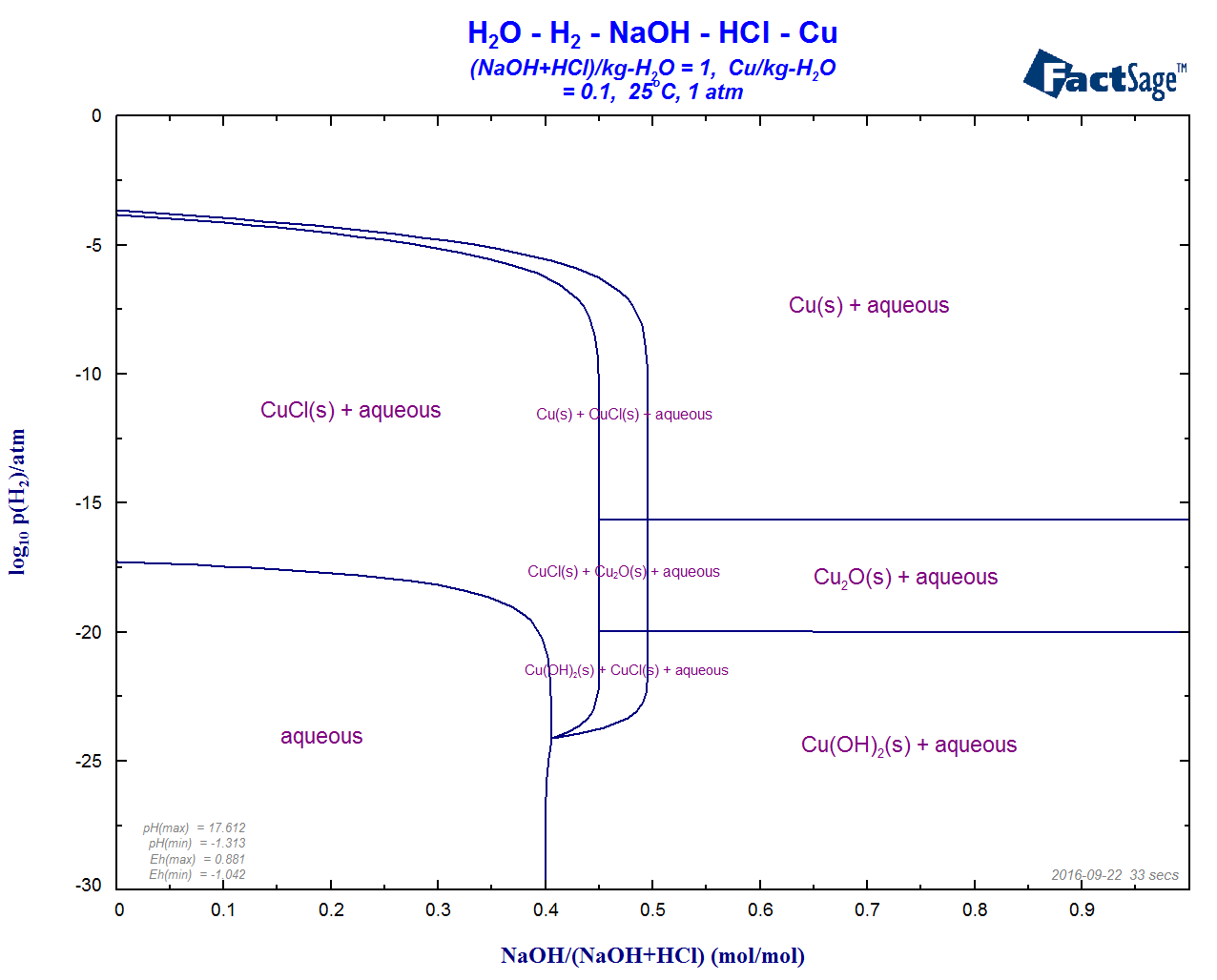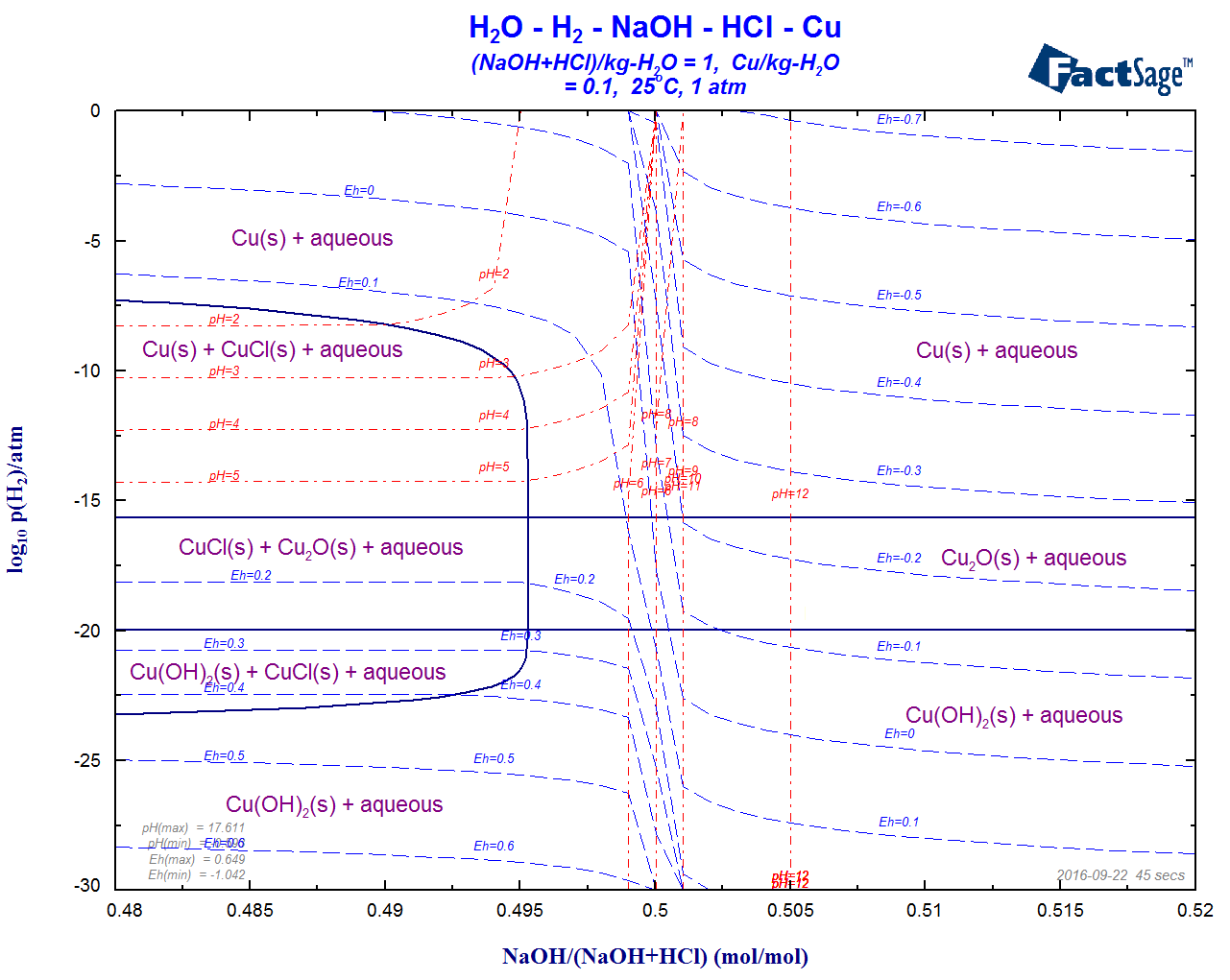Aqueous Phase Diagrams of the H2O-Cu-NaOH-HCl-H2 - log10(P(H2) versus molar ratio NaOH/(NaOH+HCl) at m(Cu) = 0.1.

In the diagram the X-axis is expanded around 0.5. The figure includes the calculated iso-Eh and iso-pH lines and illustrates shows the classical 'titration curve' behavior around equimolar NaOH/HCl.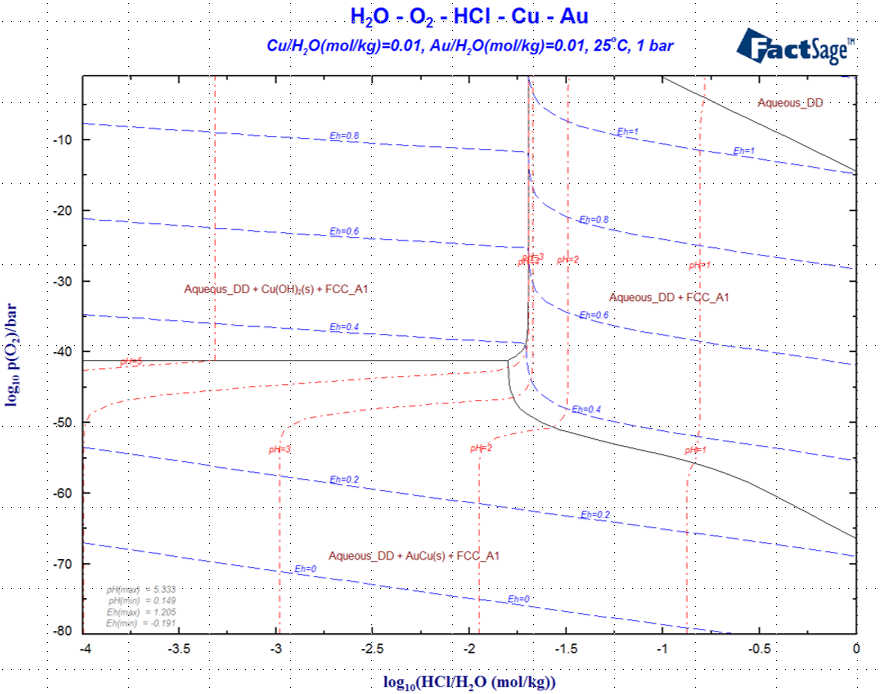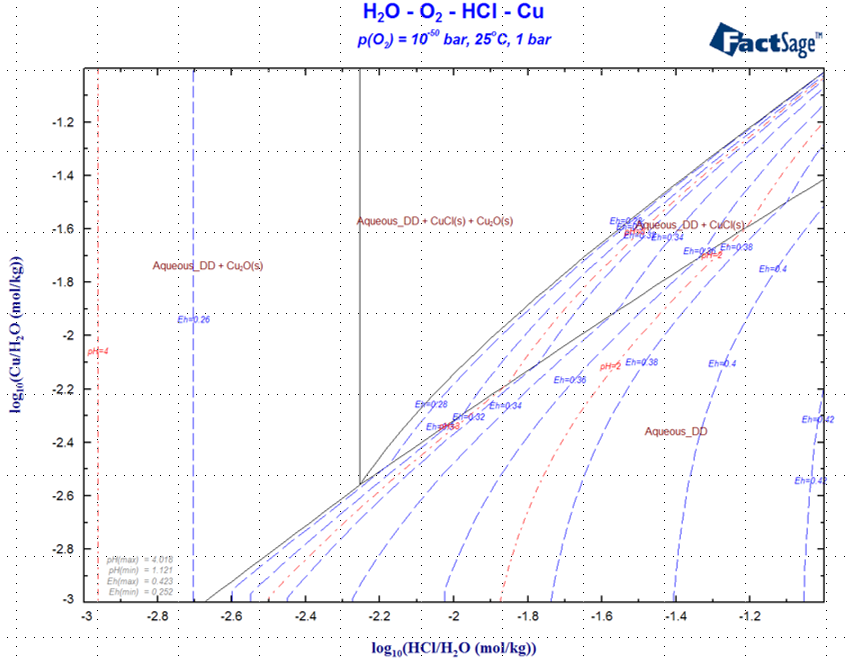Aqueous Phase Diagrams with the FThelg non-ideal aqueous solution
1 - H2O-O2-HCl-Cu-Au aqueous phase diagram involving a non-ideal alloy of Cu and Au where the y-axis is the oxidation potential, log P(O2), which is related to Eh..
2 - H2O-O2-HCl-Cu aqueous diagram with m(HCl) versus log m(Cu) at constant oxygen potential .

Details on Aqueous Phase Diagrams are given in the updated Phase Diagram Slide Show (new slides 19.1 - 19.37).

• Scheil-Gulliver Constituent Diagrams - new type of phase diagram

During cooling of an alloy from the liquid state at complete thermodynamic equilibrium, all solid solution phases remain homogeneous through internal diffusion and all peritectic reactions proceed to completion. The stable phases at various compositions and temperatures can be represented by a classical temperature versus composition phase diagram for example as calculated by the Phase Diagram module.

In practice, conditions for cooling often approach more closely to those of Scheil-Gulliver cooling in which solids, once precipitated, cease to react with the liquid or with each other, there is no diffusion in the solids, the liquid phase remains homogeneous, and the liquid and surfaces of the solid phases are in equilibrium.

The Scheil-Gulliver Constituent Diagram is a new type of phase diagram in FactSage 7.1 that we have developed for displaying the cooling path when a system is cooled and the constituents in the phases are not allowed to diffuse.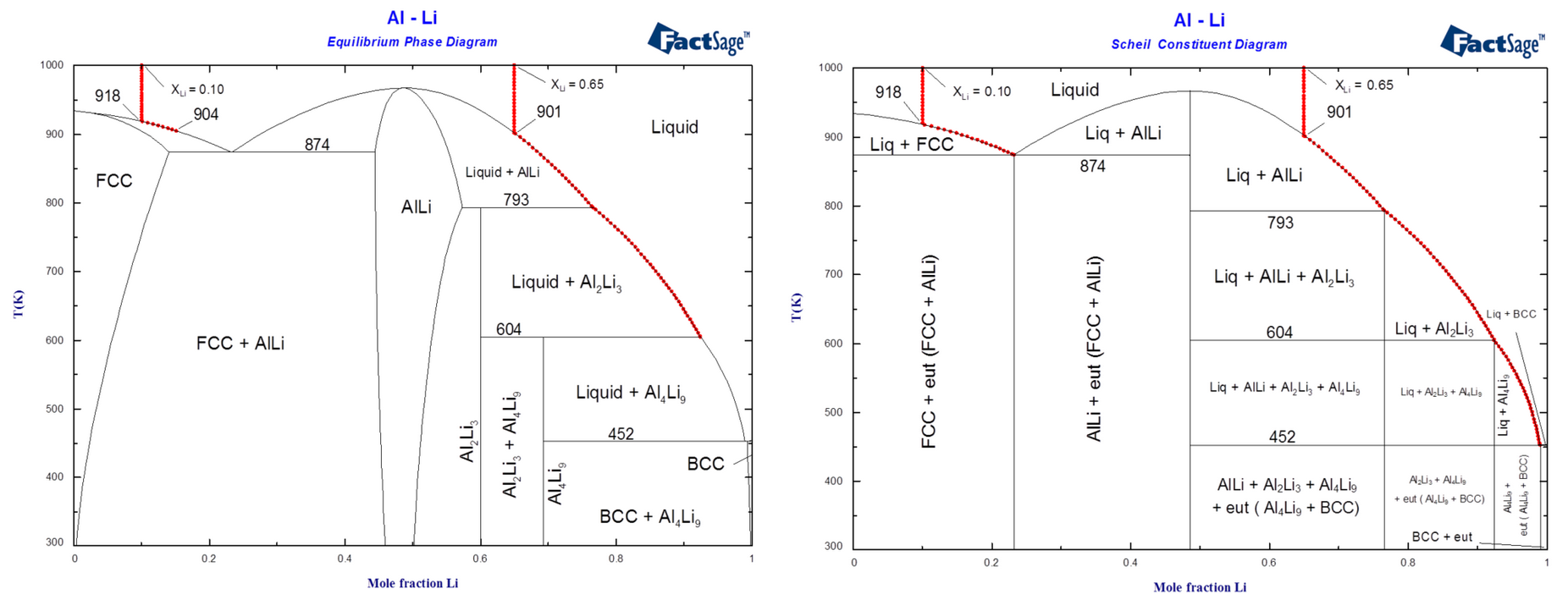Diagrams of the Al-Li system illustrating solidification of alloys of compositions XLi = 0.10 and XLi = 0.65.
1 - Calculated phase diagram and equilibrium solidification.
2 - Calculated Scheil-Gulliver constituent diagram and Scheil-Gulliver solidification.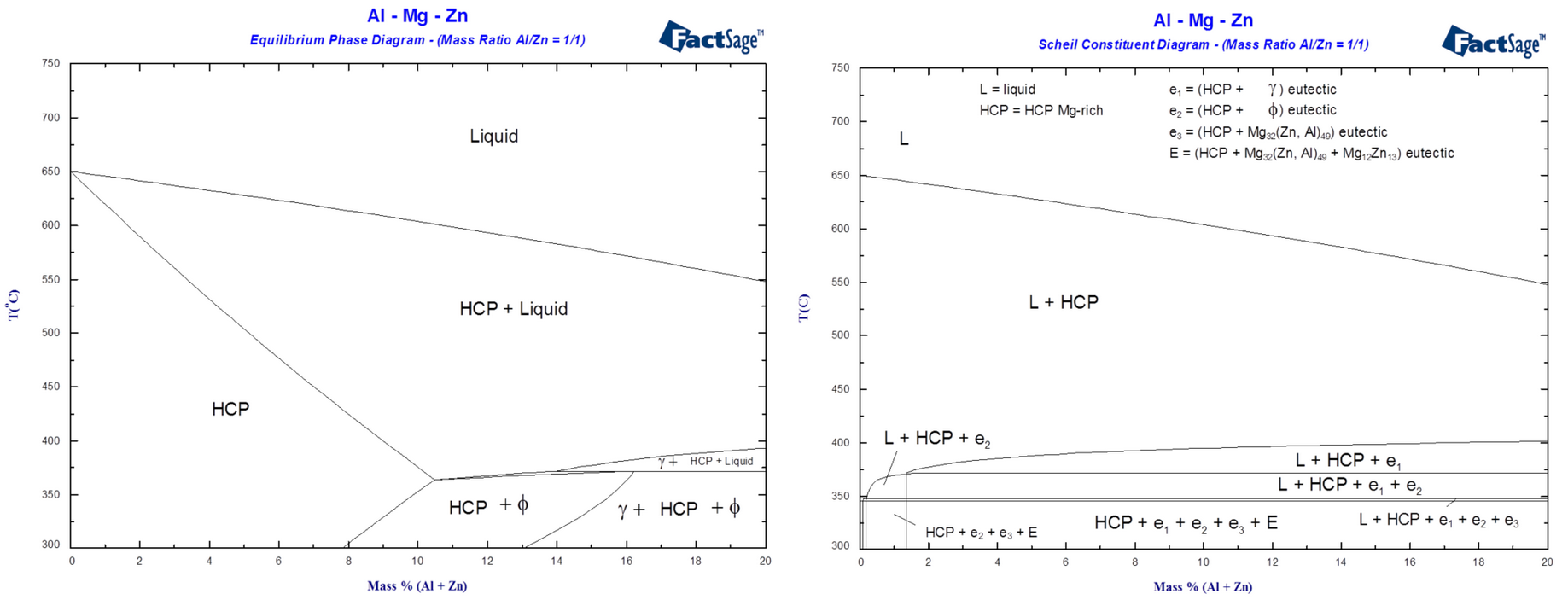Diagrams of the Al-Mg-Zn ternary system for Mg-rich alloys at constant mass ratio Al/Zn = 1/1
1 - Calculated phase diagram and equilibrium solidification.
2 - Calculated Scheil-Gulliver constituent diagram and Scheil-Gulliver solidification.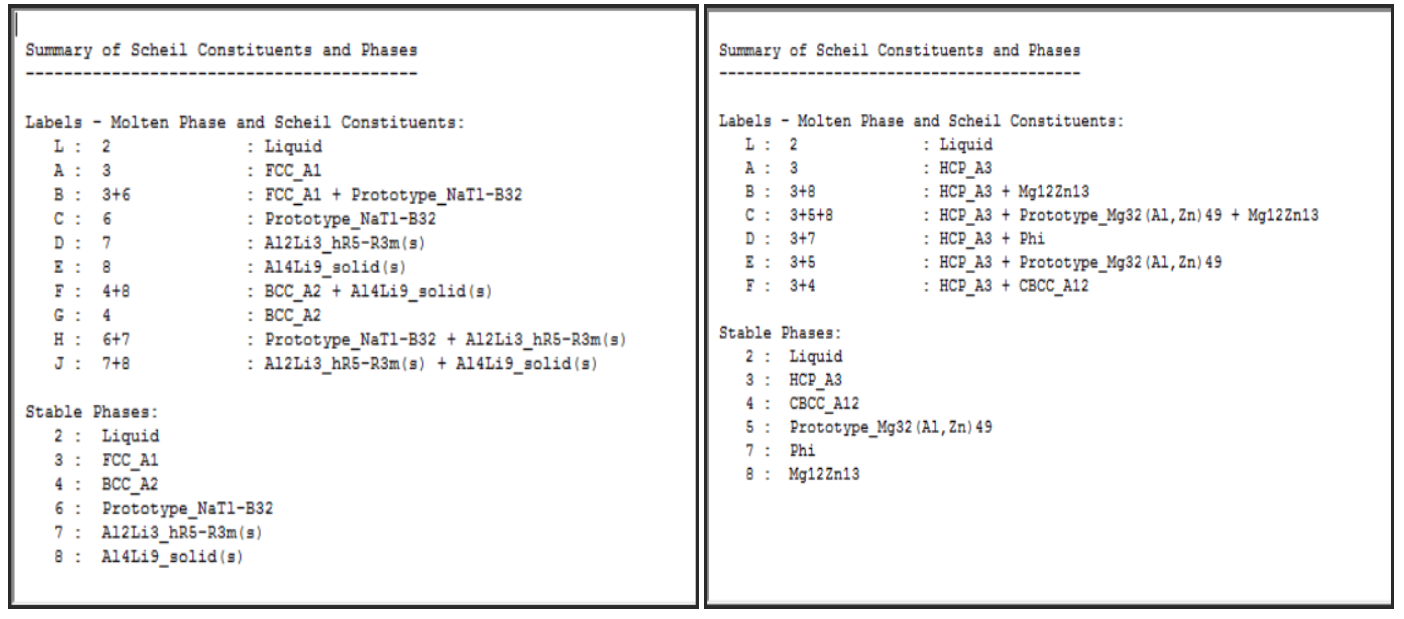Tables listing the Scheil constituents and phases during Scheil cooling.
1 - Scheil cooling in the Al-Li binary system.
2 - Scheil cooling in the Al-Mg-Zn ternary system..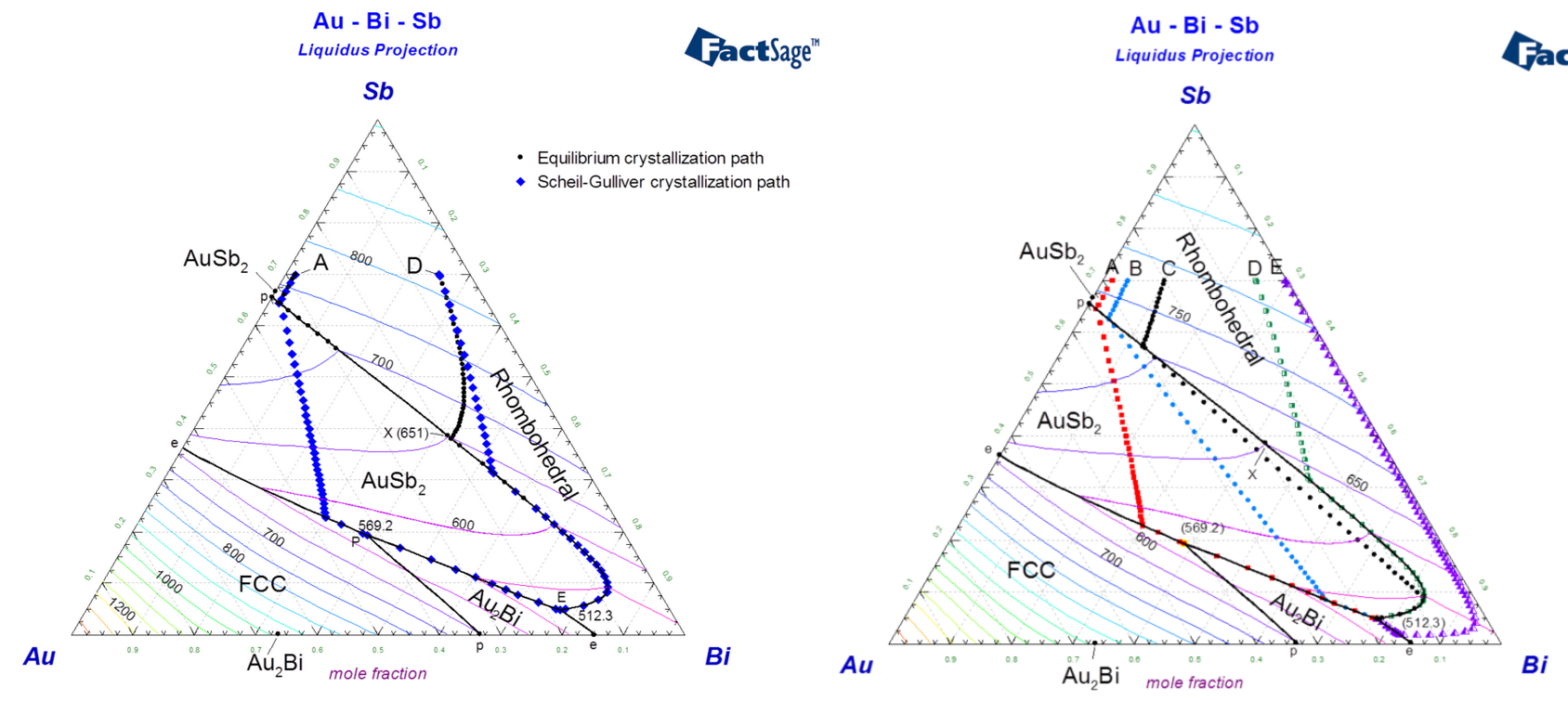Calculated liquidus projection of the Au-Bi-Sb ternary system.
1 - Crystallization paths during equilibrium cooling (circles) and Scheil-Gulliver cooling (diamonds) are shown for two alloys (A and D).
2 - Crystallization paths during Scheil-Gulliver cooling are shown for five alloys (A, B, C, D, E)

Details on Scheil-Gulliver Constituent Diagrams are given in the updated Phase Diagram Slide Show (new slides 18.1 - 18.20) as well as in a recent publication Scheil-Gulliver Constituent Diagrams by A.D. Pelton, G. Eriksson and C.W. Bale submitted to Met. Trans B, September 2016 (click on 'Slide Show > Phase Diagram - Article - Scheil-Gulliver Constituent Diagrams pdf' )

4 Phase Diagram Parameters Window - 'Phase Diagram > Menu Window > Parameters ...'

• Show options

In the Parameters Window the Show options have been enhanced.

After the phase diagram has been calculated:

- the status box now displays Information on the Stable Phases

- the new date and elapsed time options post these values in the lower right corner of the figure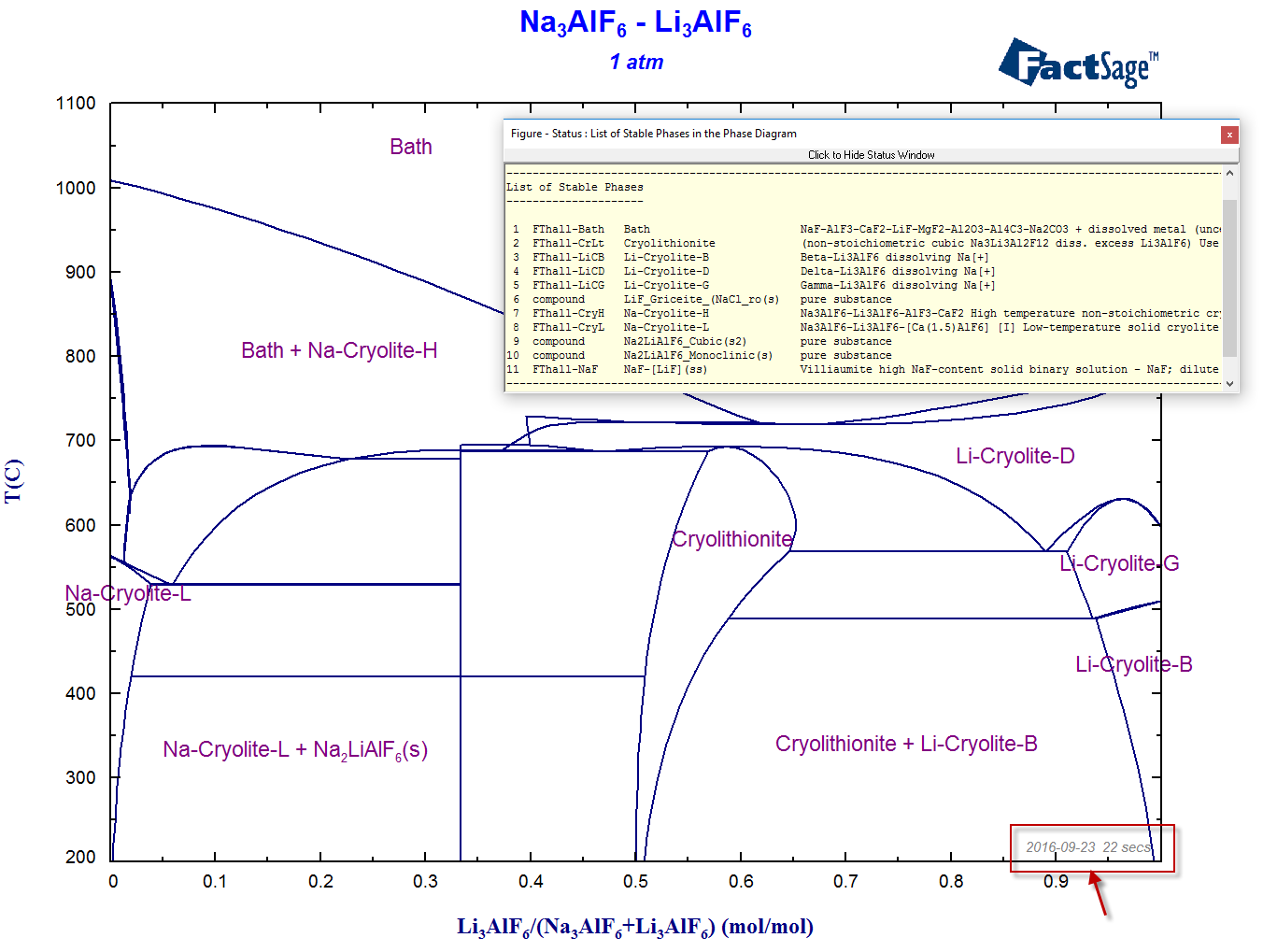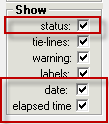The calculated Na3AlF6-Li3AlF6 phase diagram displaying the status box with 'Information on the Stable Phases'
as well as the date and elapsed time (22 secs) of the calculation .

• Labels & Lines options

In the Parameters Window the Labels & Lines options enable you to label the phases by numbers. This is useful in systems containing many phases.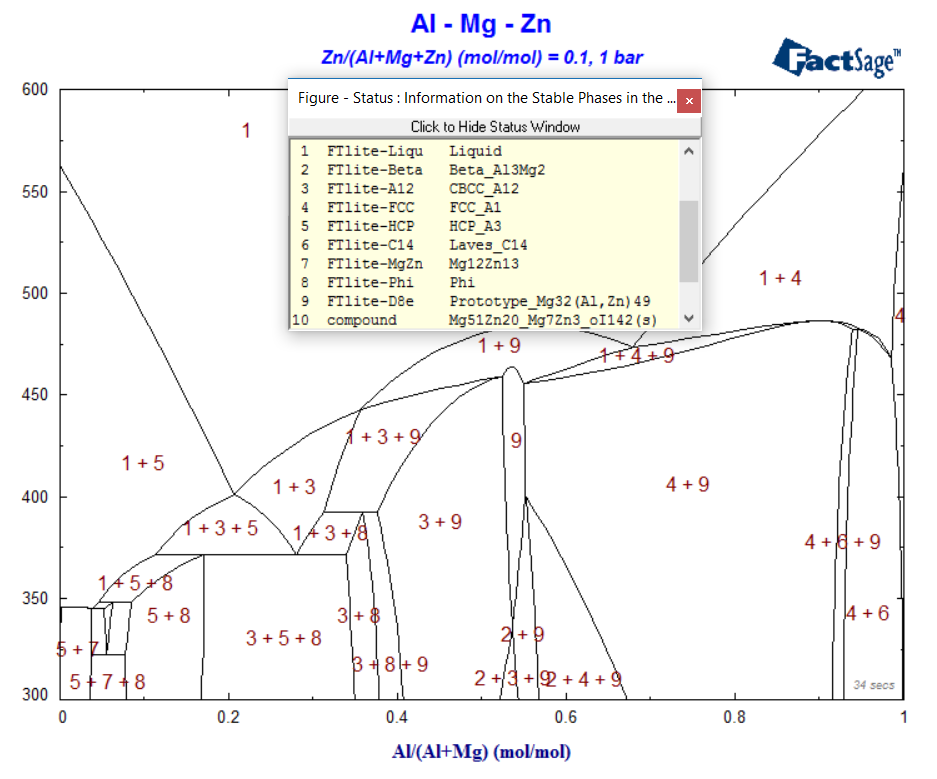Calculated T versus Al/(Al+Mg) at constant Zn in the Al-Mg-Zn system with numerical labels for the phases.
The correspondence between phase number and phase name is given in the 'Information on the Stable Phases'.

• Stop Window

 You activate the 'Stop Window' via the Parameters Window. This enables you to terminate a phase diagram calculation at any time without terminating or quitting the Phase Diagram (or Equilib) module.. In FactSage 7.1 there are some new features in the Stop Window: - The Stop Window includes the total elapsed time in seconds as well as hr-min-sec. - The current calculation step (106) and step elapsed time (11.92 sec) are posted in the window - these are taken from the Figure Status Window. - the Stop icon on the tray at the bottom of the screen is distinctive and improves readability.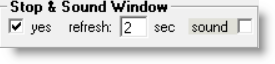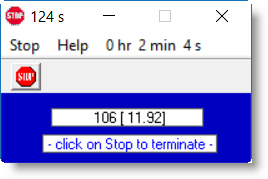• Figure and the Stop button

- When the 'Stop' is pressed in the Stop Window, the program terminates the phase diagram calculation as soon as possible.

- When done it posts the message 'Stop button pressed' in the Status Window and also adds the text '~ Stop button pressed ~' at the bottom of the figure.

- The figure stays active - that is you can still add labels, edit text and perform detailed point calculations in the usual manner.

- When done you can save the Phas*.dat file and its (incomplete) calculated phase diagram in the Menu Window in the usual manner.

FactSage 7.2 (2018)

FactSage 7.3 (2019)

• What's New in Phase Diagram - 'Phase Diagram > Components Window > Help > What's New in Phase Diagram ...'

 The new link What's New in Phase Diagram gives a summary of the more important programming changes in Phase Diagram that have appeared since FactSage 6.1 (2009).• Phase Diagram Slide Show

The slide show has been updated.

The slides which have been either modified or added are 13.5, 15.1, 19.1 (bibliographic references inserted), 19.38 and 19.39 (to show how to re-plot aqueous diagrams in Eh-pH coordinates) and Appendix 4.4 which was supposed to be included in FactSage 7.2 but was not.

• Scheil-Gulliver constituent diagrams - Phase Diagram Components Window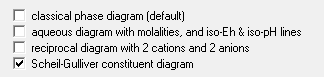In Scheil-Gulliver constituent diagrams the two options of FactSage 7.2    ZPF: zero phase fraction lines, and    ZCF: zero constituent fraction lines have been dropped for a simplified single option as shown.

• Selection of solution phase - changing [J] to [I], [I] to [+]

 The image shows the solution phase selection in the Ni-Zn system with data taken from FTlite. There are four 2-phase immiscibility [I] and two 3-phase immiscibility [J] selections for a total of 14 solution phases with 28 species. In FactSage 7.3 it is possible to change all 3-phase immiscibility [J] to 2-phase immiscibility [I] selections by clicking Select as shown.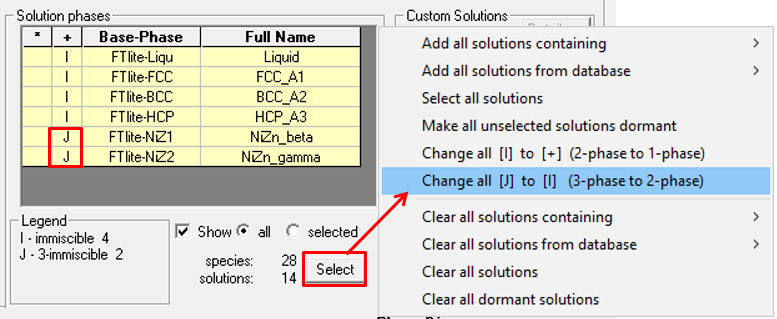The result is six 2-phase immiscibility [I] slections for a total of 12 solution phases with 24 species. In addition you can to change all 2-phase immiscibility [I] to single phase [+] selections. The result (not shown here) is 6 single phases [+] with 12 species.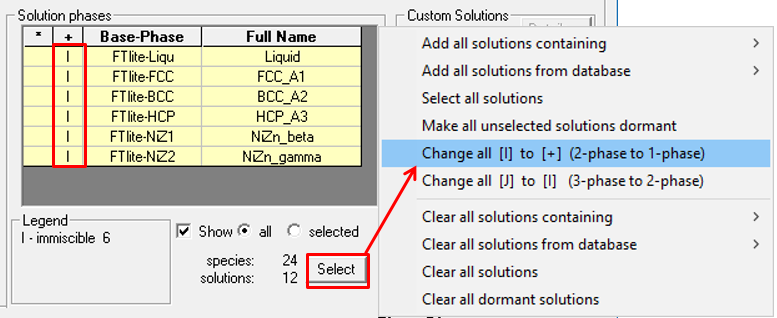• Transitions

In temperature versus composition phase diagrams a new option for phase diagram transitions has been added to the phase equilibrium mode.

The figure below is the classical T(K) vs X(Sn) binary phase diagram for Cu-Sn using data from FTlite.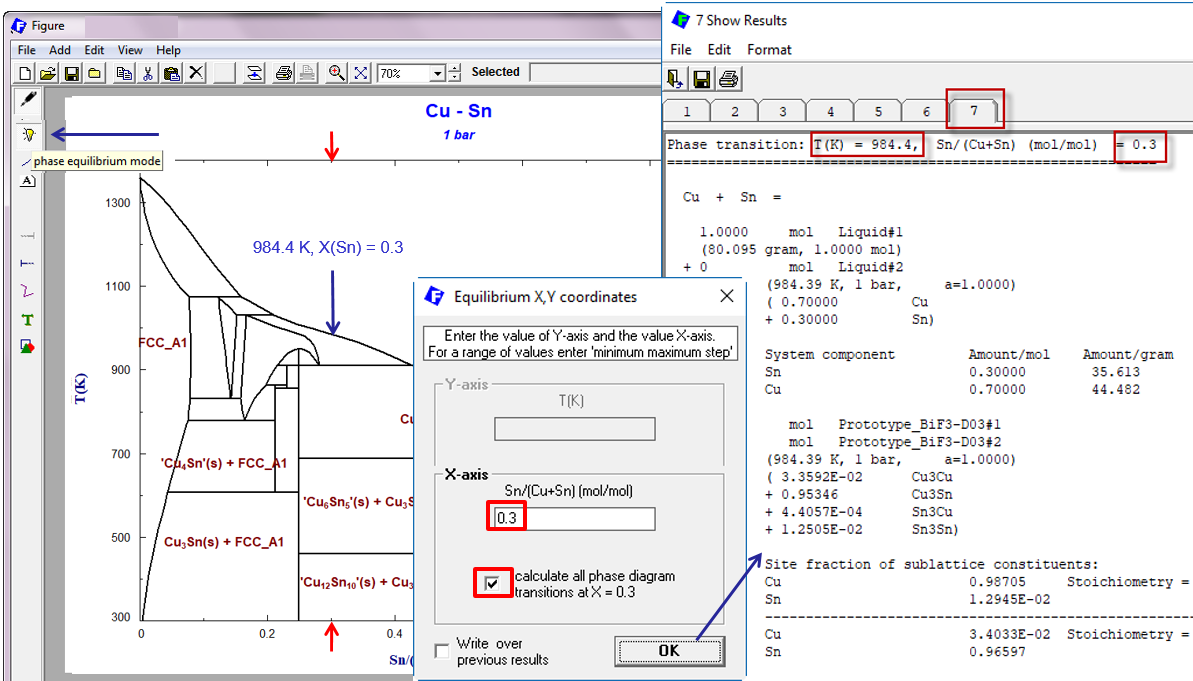After selecting the phase equilibrium mode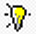, and clicking on the figure, you enter the temperature and composition in the usual manner. In FactSage 7.3 you now have the option to calculate all phase diagram transitions at the defined composition.

In the above example the Show Results Window posts all the calculated transitions at X(Sn) = 0.3. For example, the results at page 7 correspond to the liquidus temperature and composition (984.4 K, X(Sn) = 0.3) .

• List of Stable Phases

During the calculation the Status Window appears and displays information on the Stable Phases. Sometimes this happens before the diagram has been completely calculated. Uncheck the 'status' check box (see 'Phase Diagram > Menu Window > Parameters') if you want to hide the Status Window until after the calculation is finished.

 In FactSage 7.3 the information on the Stable Phases has been enhanced, particularly with respect to the compounds (pure substances PS) This is shown in the screenshots for the calculated Mn-Si binary phase diagram with data taken from FTlite,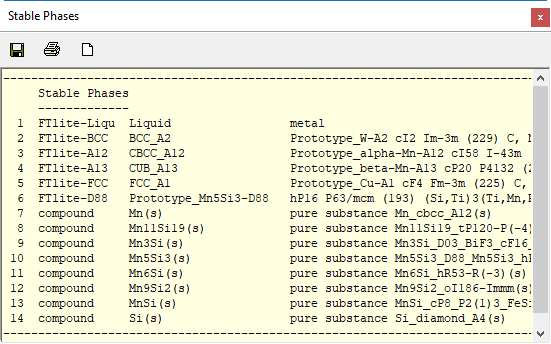FactSage 7.2FactSage 7.3

• Phase Diagram Manipulate and Refresh Window

 The Phase Diagram Manipulate and Refresh Window was first introduced in FactSage 7.2. For complete details click Phase Diagram Manipulate and Refresh Window. The screenshot shows how to activate the Manipulate and Refresh Window via the Parameters Window ('Phase Diagram > Menu Window > Parameters')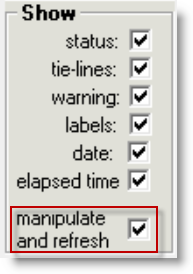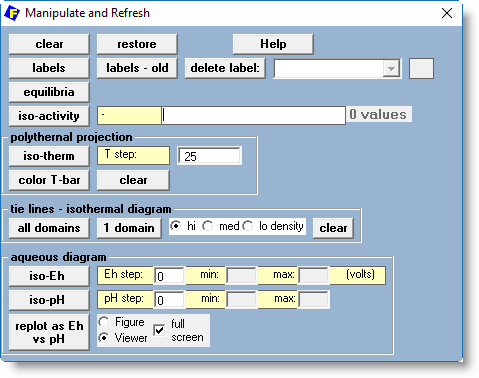The Phase Diagram Manipulate and Refresh Window is a popular application that offers a variety of editing and plotting options depending upon the type of calculated phase diagram. It is displayed right after the phase diagram has been calculated. The Window offers 4 categories for manipulating the calculated phase diagram: General Polythermal projection Tie lines Aqueous diagram Note - when you display a stored phase diagram, e.g. show the figure calculated last time - most of the categories (except 'labels - old') are not available.

• General

• labels - old - this is new in FactSage 7.3

When loading a previously stored system, you can show the figure calculated last time. The program displays the calculated phase diagram including any labels that have been added manually. These labels are now stored in memory. If you return to the Menu Window, make changes to the phase selections, axes limits, etc. and recalculate the diagram, the new figure will not have any labels. By clicking labels - old the labels that were stored in memory are posted.

• Aqueous diagram

These options only apply to aqueous diagrams.

• iso-Eh - plots iso-Eh(volts) on the aqueous diagrams. The step in Eh and the limits min. and max. can be redefined in the text boxes.

• iso-pH - plots iso-pH lines on the aqueous diagrams. The step in pH and the limits min. and max. can be redefined in the text boxes.

Min. and max. are new in FactSage 7.3. These text boxes mean that you are no longer obliged to always the plot the (time-consuming) iso-Eh and iso-pH lines. These lines can simply be added afterwards whenever needed.

FactSage 8.0 (2020)

• New Phase Diagram file structure - *.phas

 Time limit - Phase Diagram Parameters Window     - refer to Time limit - Equilib Parameters Window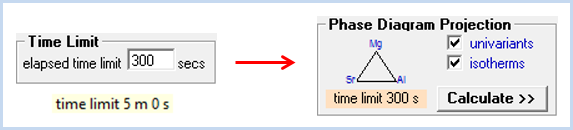• Automatic temperature labels on polythermal projections

In FactSage 8.0 there is a new option to automatically label the temperatures of the iso-therms on a polythermal projection.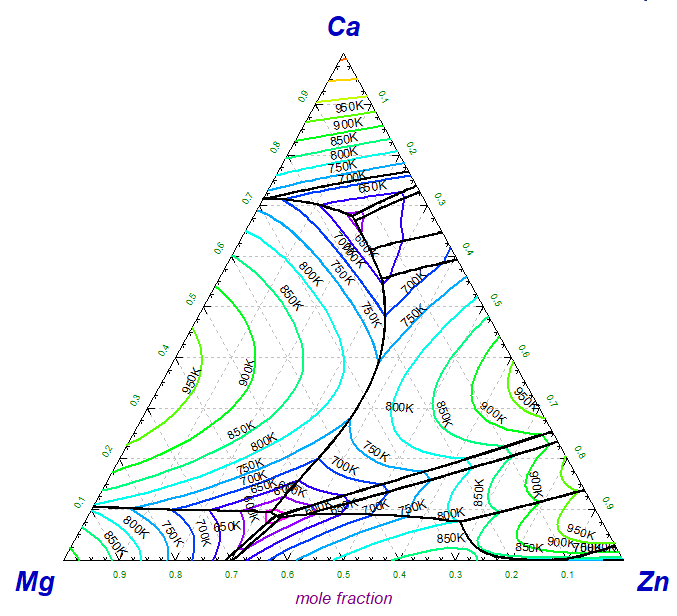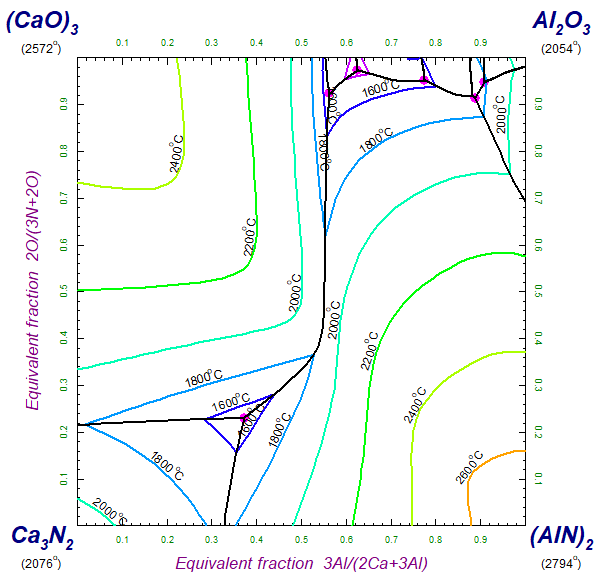The screenshots show the polythermal projections of the Ca-Mg-Zn ternary alloy system and Ca-Al-N-O ternary reciprocal system taken from the stored examples - Phase Diagram > Help > Directory of examples > Polythermal Projections ....'

The temperature labels (together with the optional units K or oC) have been plotted automatically. They are aligned with the isothermal lines and displaced slightly to improve readability.

How the temperature labels are generated is shown next in the Manipulate and Refresh Window.

• Phase Diagram Manipulate and Refresh Window

 For complete details and examples click Phase Diagram Manipulate and Refresh Window. The Phase Diagram Manipulate and Refresh Window was first introduced in FactSage 7.2, enhanced in FactSage 7.3 and again here in FactSage 8.0. The screenshot shows how to activate the Manipulate and Refresh Window via the Parameters Window ('Phase Diagram > Menu Window > Parameters')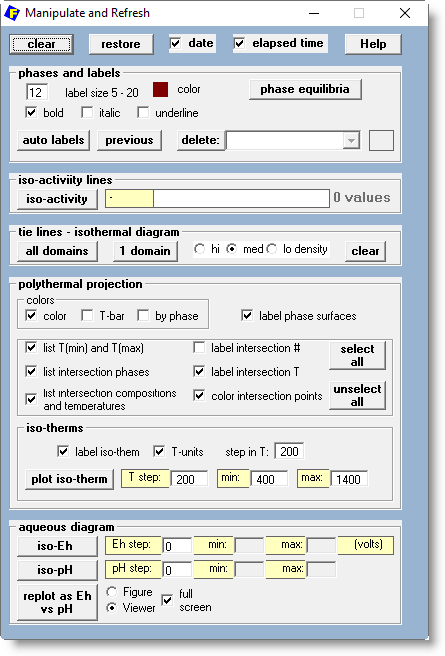In addition to the General options that apply to all diagrams, there are now 5 categories for manipulating the calculated phase diagram: phases and labels iso-activity lines tie lines - isothermal diagram polythermal projection aqueous diagram The screenshot shows all 5 categories but in actual calculations only 'phases and labels' is displayed together with the other pertinent option. For example, only one of 'tie lines - isothermal diagram', 'polythermal projection' or 'aqueous diagram' could be displayed at any given time.

The screenshots below show the Manipulate and Refresh Window for a polythermal projection.

In FactSage 8.0 the effects of the options 'color', 'label iso-therms', 'label phase surfaces', 'list T(min) and T(max)', etc. are now stored when the diagram is first calculated. With the Manipulate and Refresh Window you then select which options you wish to apply - the phase diagram is not recalculated but rather it is redisplayed. This is different from FactSage 7.3 where changing the polythermal projection display options in the Parameters Windows would necessitate a recalculation of the diagram.

 In the left diagram most of the display options are unchecked - click 'unselect all'.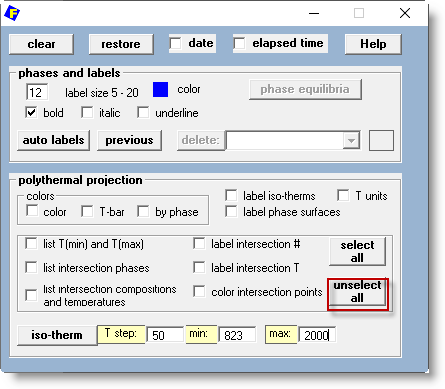In the right diagram the color and display options are all checked - click 'select all' - as well as the labels.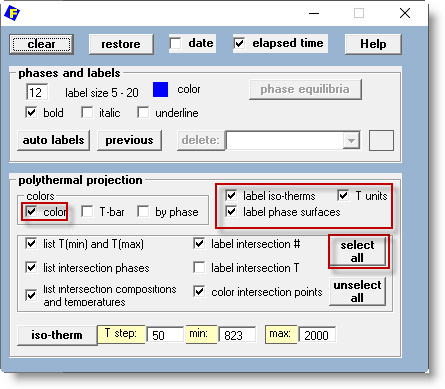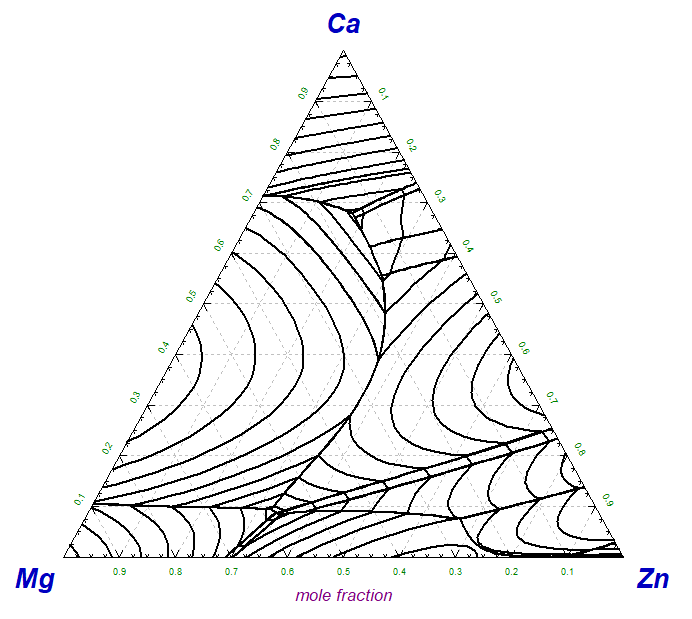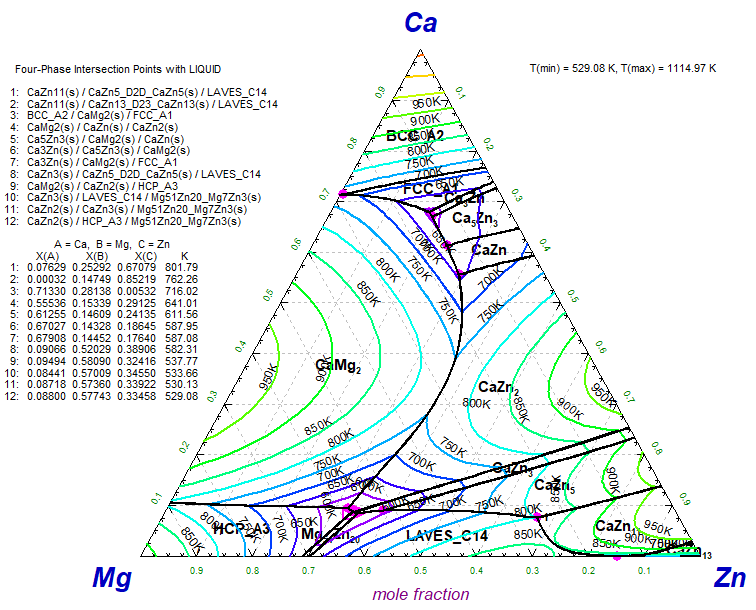For more details and examples click Phase Diagram Manipulate and Refresh Window.

FactSage 8.1 (2021)

[www.FactSage.com] [CRCT] [GTT]